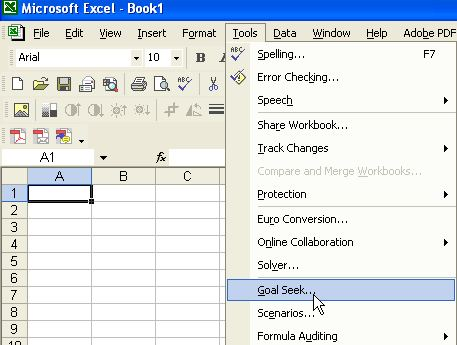Share this: Google+

## How to use Goal Seek in Excel?

Microsoft Excel has ability to do inverse problem using Tools-Goal Seek . Goal Seek is simple to use and proficient to solve equations with single unknown variable. If you need to solve several unknown simultaneously, you may need more powerful tool: MS Solver.

The fololwing tutorials will demonstrate several applications of Goal Seek . You may free download the full set of spreadsheet examples here .

Click any topics below to enter the particular tutorial:

Find Real Root of Polynomial Equation using MS Excel
Determination of product price based on Break Event Point
Obtaining zeros of non-linear equation

Solving inequality using MS Excel Goal Seek
Finding Eigen value of Symmetric matrixPreferable reference for this tutorial is

Teknomo, Kardi (2015). Microsoft Excel Goal Seek . https:\\people.revoledu.com\kardi\ tutorial\Excel\GoalSeek.html